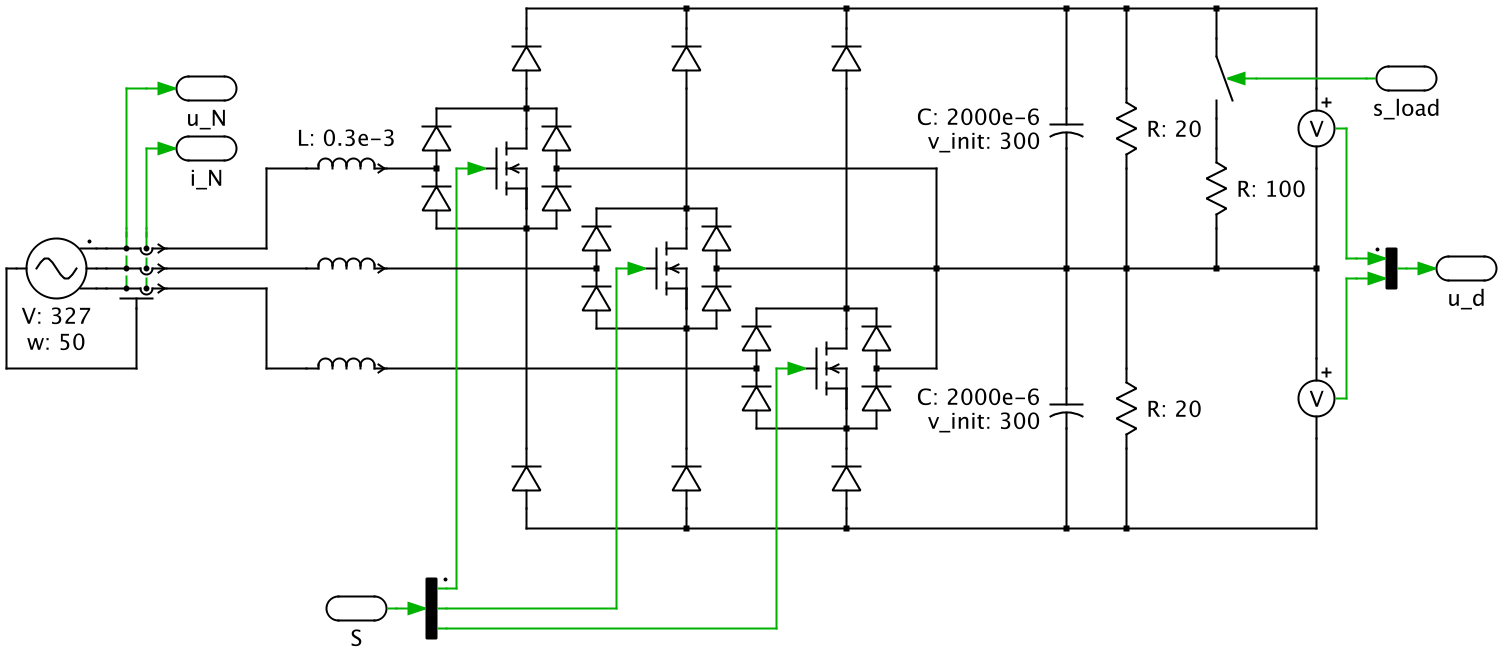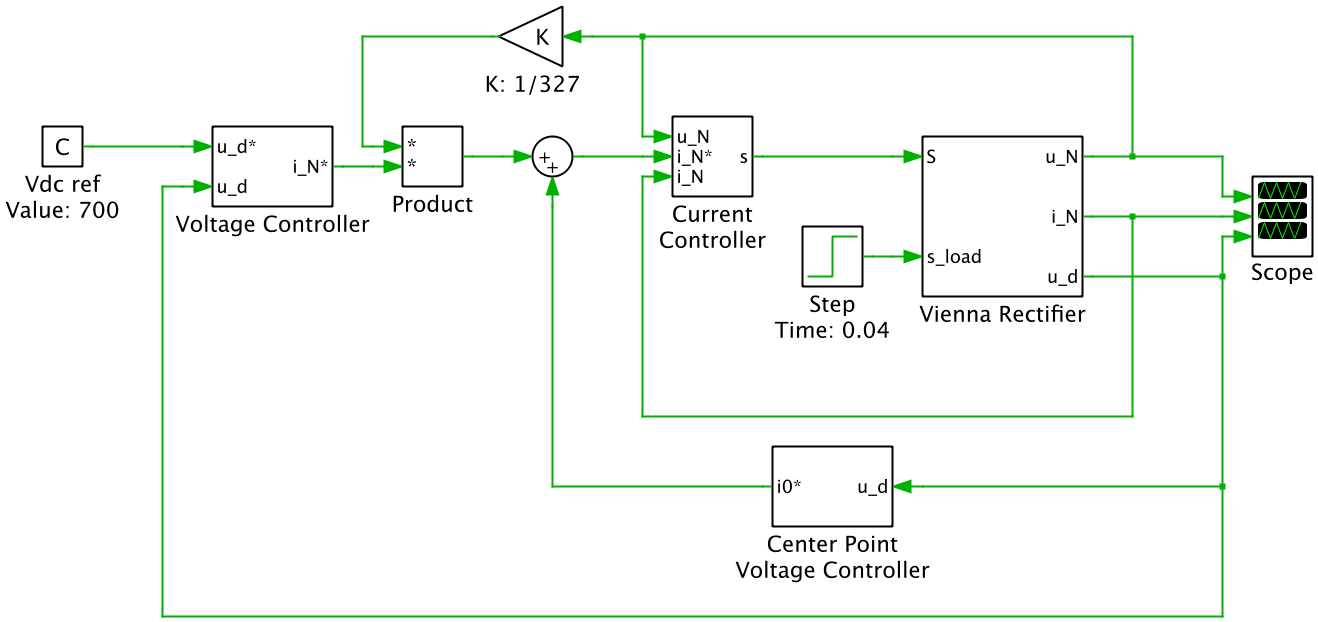# Vienna Rectifier with Hysteresis Current Controller

This PLECS demo model shows a Vienna Rectifier with an output voltage of 700 V and an output power of 12.25 kW. The simulation combines the electrical power circuit and the cascaded controls.

### Power circuit

The Vienna Rectifier is a unidirectional three-phase three-switch three-level PWM AC-DC converter:### Control

The controls are modeled with functional subsystem blocks. The controls are cascaded and consist of a current loop, a DC center point voltage loop and a DC voltage loop.The outermost DC voltage loop regulates the sum of the two capacitor voltages using a PI controller. The controller output is the reference for the mains current amplitude IN*. This amplitude is multiplied with a three-phase sinusoidal signal which is synchronized with the mains voltages in order to obtain the three-phase mains current reference iN*. For simplicity, this sinusoidal signal is generated directly from the measured mains voltages.

The mains current reference signal is offset by a DC current reference i0*. This DC reference is the output of a PI controller regulating the difference of the two capacitor voltages.

Finally, the mains current reference signal is used by the hysteresis current controller to generate the gate signals for the semiconductor switches. Due to the operating principle of the Vienna Rectifier, the gate signal of an individual phase needs to be inverted during the negative half-wave of the corresponding mains phase voltage.

### Simulation

The simulation shows the controller response to a sudden asymmetrical loading of the output voltage. The scope shows the sinusoidal mains voltage, the hysteresis controlled mains current and DC voltages of the two output capacitors.

At simulation start, the capacitors are charged from their initial 300 V to their nominal level at 350 V. At t=0.4 s, the load becomes unbalanced. Without the center point voltage controller, the capacitor voltages would also quickly become unbalanced. With the controller in operation there is only a small deviation, and the capacitors are balanced again after about 0.1 s.

### Try it

This model is available in the PLECS Demo Model library provided in both versions of PLECS.

Categories: